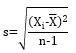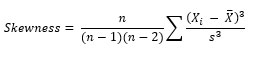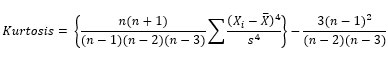June 2017

(Note: all the previous publications in the basic statistics category are listed on the right-hand side.  Select "Return to Categories" to go to the page with all publications sorted by category.  Select this link for information on the SPC for Excel software.)Software today will easily generate descriptive statistics for a set of data.  These descriptive statistics, as the name implies, are supposed to “describe” the data.

This is the first thing some people do.  We take samples for lots of reason.  Sometimes we are trying to estimate some population parameter such as the average or standard deviation.  Other times we just want to know something about a particular sample and don’t care about anything beyond that sample.

There can be lots of descriptive statistics generated by the software.  Some of these statistics are familiar, such as the count, average, and standard deviation.  Others may not be so familiar, such as the coefficient of variation and standard error.   This month’s publication looks at the typical descriptive statistics and what they mean.

In this issue:

### Example Data

Suppose we have taken 25 observations from a process.  The 25 observations are given in the table below.

Table 1: Our Data

 104.1 87.6 104.3 97.9 101.6 97.3 101.8 104.1 94 94 93.5 106.9 77.4 106.6 114.2 100.3 104.1 97.6 98.4 97.8 102.8 101.2 85 115.1 108.3

We will use these data to generate some descriptive statistics and then look at how they are calculated and what they mean.

### Software Output

The descriptive statistics output from the SPC for Excel software is shown below (not including the histogram and dot plot options).  The next section takes a look at what each statistic means and how it is calculated.  The table rounds some values.

Table 2: Descriptive Statistics for Our Data

 Mean 99.84 Standard Error 1.684 Mode 104.1 Standard Deviation 8.421 Variance 70.91 Coefficient of Variation 8.434 Kurtosis 1.23 Skewness -0.678 Range 37.7 Minimum 77.4 Maximum 115.1 Sum 2495.9 Count 25 First Quartile 97.3 Median 101.2 Third Quartile 104.1 95% Lower Conf. Limit 96.37 95% Upper Conf. Limit 103.3

### Descriptive StatisticsThis section explains the output shown in Table 2.

Average, Standard Deviation and Variance

The two most common statistics are the mean and the standard deviation.  The average we all seem to understand – or do we?  The average or mean( X) is defined as the following:

X=∑Xi/n=  2495.9/25=99.836

where Xi is an individual observation and n is the number of observations.  The AVERAGE function in Excel does this calculation. This is the true average of the sample.

Sometimes we make the mistake of taking a sample – maybe even a large sample – and calculating the average.   Then we assume that this the average of the process.  It is the average of the sample – but maybe nowhere near the average of your process.  One of our previous publications: - When an Average Isn’t The Average – examines this possibility.

The standard deviation (s) is not as well understood as the average by most people.  It is a measure of variation in the observations in the sample.  The larger the standard deviation, the more variation in the observations.  The smaller the standard deviation, the less variation in the observations.  The standard deviation of the observations is given by:The standard deviation in our data is 8.421.  The STDEV function in Excel will calculate the standard deviation.

Explaining the Standard Deviation is an earlier publication that explores how the standard deviation is calculated, what it means, and how it is used.  According to Google Analytics this is the 5th most viewed of our publications this year with over 14,100 views.This is the standard deviation that is estimated from the range (R) control chart (either the moving range or subgroup range).  There are three different methods that the standard deviation is estimated for use in control charts.  Please see our publication, The Estimated Standard Deviation and Control Charts for more information.  This is our 7th most viewed publication this year with about 11,400 views.  The estimated value of the standard deviation is often denoted by σ in control chart theory.

The variance is also a measure of the spread in the data.  It is calculated by squaring the standard deviation.

Standard Error of the Mean

The standard error of the mean estimates the variation in samples means.  If we took additional samples of 25 observations and calculated the sample average of each, the standard error of the mean will describe the variation in those samples means.

The larger the standard error of the mean, the more variation in the sample means – meaning a larger interval for an estimate of the true population mean.  The smaller the standard error of the mean, the less variation in the sample means – meaning a smaller interval for an estimate of the true population mean.  The standard error (SE) of the mean is given by:

SE=s/√n=  8.421/√25=1.684

Mode

The mode is the value that occurs most often. There can be multiple modes, i.e., there can be 2 or more values that occur the most.  If no value occurs more than once, there is no mode for the sample.  In the data above, 104.1 occurred the most (3 times) so the mode of the sample is 104.1.

Coefficient of Variation

The coefficient of variation (COV) is defined by:

COV=100 (s/ X )=100(8.421/99.836)=8.434

The COV is a measure of variation relative to the average.  Since it is the standard deviation divided by the average, it does not have any units.  You can use the COV to compare the variation in data sets that have different units or different averages.

Skewness and KurtosisSkewness and kurtosis are two measures of distribution’s shape.  Skewness is a measure of the symmetry in a distribution.  A symmetrical dataset will have a skewness equal to 0.  So, a normal distribution will have a skewness of 0.   Skewness essentially measures the relative size of the two tails.  Skewness is calculated from the following;This is the equation that Excel uses with its SKEW function. The skewness for the observations is -0.678.

Kurtosis is a measure of the combined sizes of the two tails.  It measures the amount of probability in the tails.  The value is often compared to the kurtosis of the normal distribution, which is equal to 3.  If the kurtosis is greater than 3, then the dataset has heavier tails than a normal distribution (more in the tails).

If the kurtosis is less than 3, then the dataset has lighter tails than a normal distribution (less in the tails).  Careful here.  Kurtosis is sometimes reported as “excess kurtosis.”  Excess kurtosis is determined by subtracting 3 form the kurtosis.  This makes the normal distribution kurtosis equal 0.  The equation for kurtosis is:This is the equation that Excel uses with its KURT equation.  The kurtosis for the observations is 1.230. Our previous publication - Are Skewness and Kurtosis Useful Statistics? - is our most view publication with over 31,600 views this year.  This publication gives a lot more information on these two statistics.

Minimum, Maximum and Range

These three statistics are straight forward.  The minimum value (77.4) is the minimum of the observations – the smallest one.  The maximum value (115.1) is the maximum of the observations – the largest one.

And the range is simply the maximum observation – the minimum value (115.1 – 77.4 = 37.7).  The range has to be greater than or equal to 0.  You can find the minimum and maximum values of observations by using Excel’s MIN and MAX functions.

Sum and Count

Two more straightforward statistics.  Sum is simply the sum of the observations – add them up.  Count is the number of observations.  You can use Excel’s SUM and COUNTA functions to sum and count the observations.

First Quartile, Median and Third QuartileThe median is the number that is in the middle of the observations.   To find the median, you can sort the data from smallest to largest.  If there are an odd number of observations, the median is the middle value.  If there are an even number of observations, the median is the average of the two numbers in the middle.  The median of the observations is 101.2

The first quartile is the median of the data less than the median.  This means that about 25% of the observations lie below the first quartile.  75% of the data lies above the first quartile.   The first quartile of the observations is 97.3.

The third quartile is the median of the data above the median. This means that about 75% of the observations lie below the third quartile and 25% of the observations lie above the third quartile.  The third quartile is 104.1

Excel uses the method of interpolation to determine quartiles.  This was incorrectly stated when this publication was first published.  Please see the note in the comments section below.

It should be noted that there are other methods of determining the quartiles that give slightly different answers.  This article from Wikipedia describes three methods.

95% Confidence Limits

The 95% confidence limits are for the average.  If you took 25 observations 100 times, 95% of the time the confidence interval defined by the confidence limits will contain the true population average.   The equation for is:

Upper Confidence Limit=  X+t(SE)

Lower Confidence Limit=  X-t(SE)

where t is the value of the t distribution for alpha = 0.05 and the degrees of freedom associated with the sample size.  The degrees of freedom is equal to n – 1 for the sample.  You can use the TINV function in Excel to find the t value.  TINV(0.05, n-1) = 2.604 for n = 25.  The average and standard error of the mean can then be inserted into the formulas above to find the confidence limits of 96.37 to 103.3.

### Summary

This publication has looked at some of the typical descriptive statistics that are available with software today.  Each statistic was explained along with how to calculate the statistic.

value than the one you got from the experiment.  If the p-value is greater than alpha, you accept the null hypothesis.  If it is less than alpha, you reject the null hypothesis.

SPC for Excel Software

SPC Training

SPC Consulting

Ordering Information

Thanks so much for reading our publication. We hope you find it informative and useful. Happy charting and may the data always support your position.

Sincerely,

Dr. Bill McNeese
BPI Consulting, LLC#### Connect with Us

•Bill, I've got bad news. Microsoft still has quirky quartiles. Neither their .INC or .EXC quartiles consistently follow the simple median inclusion and exclusion rule that you describe. The problem arises with even sample counts, which we might think would be simpler than odd.For instance, consider {1,2,3,4}. The 1st Quartile is obviously 1.5 and the 3rd Quartile is obviously 3.5 by either rule, right? Unfortunately Excel doesn't think so, because it uses interpolative quartiles and not truly inclusive or exclusive quartiles.

Jul 03, 2017
•Yes, you are correct.  Excel does have quirky quartiles. The calculation of quartile has changed in Excel through difference versions.  It is not based on the medians that this publication originally implied.  I changed the article to reflect this.  Simply a case of taking one example, applying the media approach, and having it work - then assuming it was correct.

Thanks as usual.

Jul 03, 2017

### Filtered HTML

• Allowed HTML tags: <a> <em> <strong> <cite> <code> <ul> <ol> <li> <dl> <dt> <dd> <h1> <h2> <h3> <h4> <h5> <h6> <img> <hr> <div> <span> <strike> <b> <i> <u> <table> <tbody> <tr> <td> <th>
• Lines and paragraphs break automatically.

### Plain text

• No HTML tags allowed.
• Lines and paragraphs break automatically.
This question is for testing whether you are a human visitor and to prevent automated spam submissions.

#### SPC Knowledge BaseSign up for our FREE monthly publication featuring SPC techniques and other statistical topics.